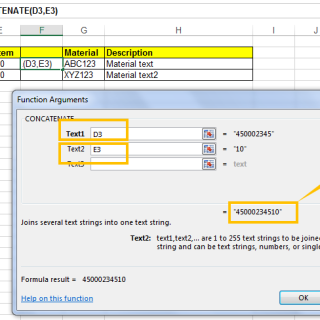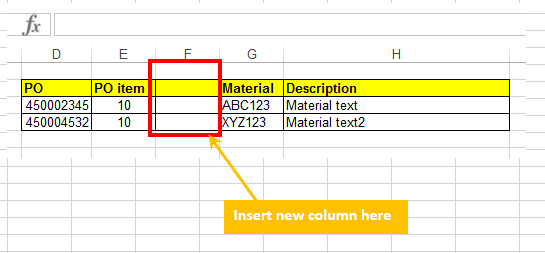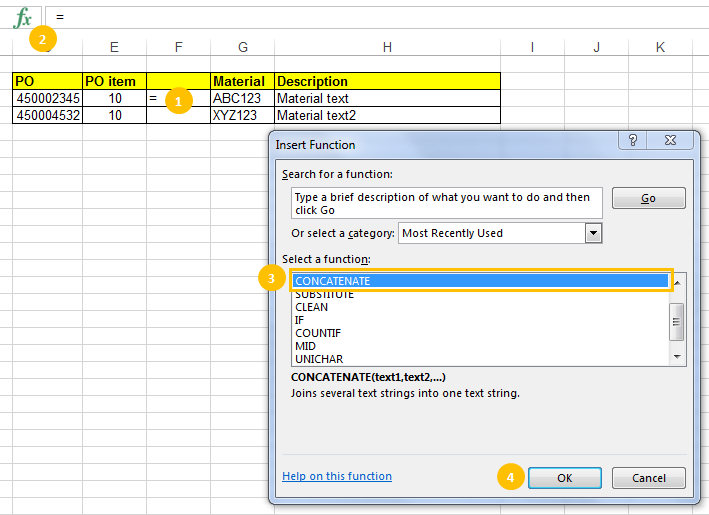# Concatenate function in MS Excel

Concatenate Function in MS Excel

Concatenate function in MS Excel is an important and simple function, which can be learned easily. It is used to join two or more strings (Text) to make one string.

Let us understand the syntax first:text1: It is a mandatory field. Here we can enter the item (text value, number, or cell reference) which we want to join.

text2: Here we can enter the second item to join.

It would be interesting to know that we can enter up to 255 items, up to 8,192 characters.

Now we will discuss one example to understand use of Concatenate function in MS excel.

Step 1: There is a set of data as shown in below screen shot.Step 2: Now we want to Concatenate PO and PO item cell. We will insert a new column between PO item and Material Column.Step 3: Now apply formula as shown in below screen shot.1. Click on the cell where we want to apply the formula.
2. Click on formula button. A new pop up window will appear.
3. Select the Concatenate formula
4. Click OK button to apply formula.

Step 4: A new pop up screen will appear and here we will enter necessary cell numbers as shown in below screen shot. First enter Text 1, then Text2in the result Text1 and Text 2 are joined.Press OK button and see the final result on excel sheet.Value of both cells joined.

Other then joining values of excel sheet cells. We can also join a cetain Text with the value of a cell.

For example : If we want to add a Text – WORLD after the Material (Column G). Then use this formula:

=CONCATENATE(G3,”WORLD”).

Output will be : ABC123WORLD.

Here you can notice that the additional text is between “”.

If you want to give a space between the two items then use formula in this way:

=CONCATENATE(G3,” “, “WORLD”)

Output will be : ABC123 WORLD.

### 1 Comment

•AJIT SAMBHUS February 7, 2017

This is very useful function & helps a lot
Thanks